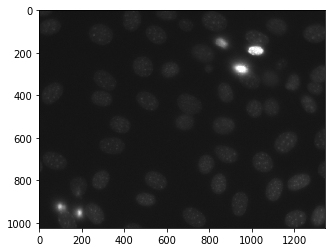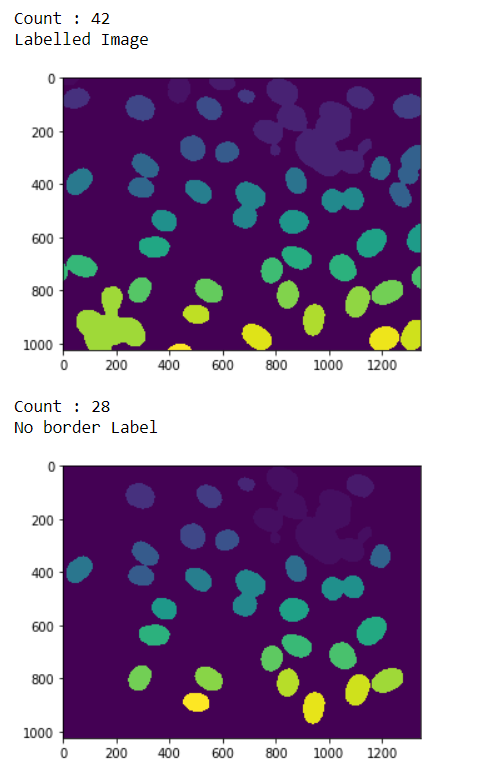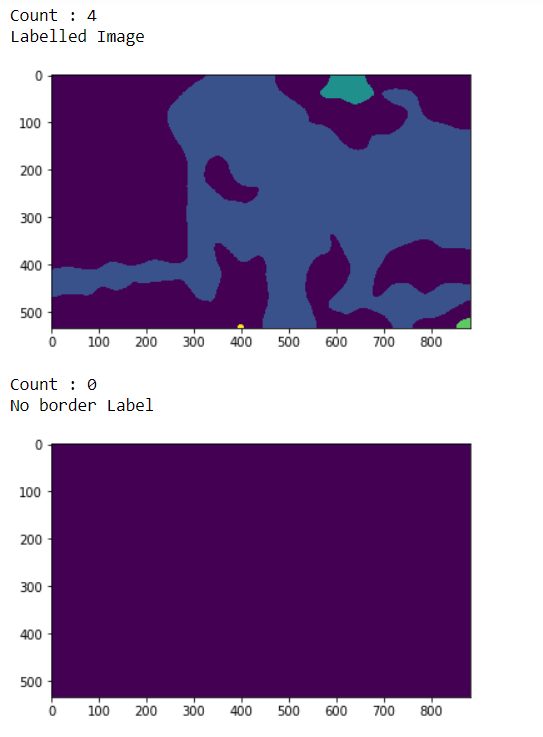# Mahotas – Re-Labeling

In this article we will see how we can relabel the labelled image in mahotas. Relabeling is used to label the already labelled image, this is required becuase some times there are mane labels which user deletes so when that image get relabel, we get the new label number as well. We use `mahotas.label` method to label the image

For this we are going to use the fluorescent microscopy image from a nuclear segmentation benchmark. We can get the image with the help of command given below

`mhotas.demos.nuclear_image()`

Below is the nuclear_imageLabeled images are integer images where the values correspond to different regions. I.e., region 1 is all of the pixels which have value 1, region two is the pixels with value 2, and so on

In order to do this we will use `mahotas.relabel` method

Syntax : mahotas.relabel(labeled)

Argument : It takes labeled image object as argument

Return : It returns the labelled image and integer i.e number of labels

Example 1:

 `# importing required libraries ` `import` `mahotas ` `import` `numpy as np ` `from` `pylab ``import` `imshow, show ` `import` `os ` ` `  `# loading nuclear image ` `f ``=` `mahotas.demos.load(``'nuclear'``) ` ` `  `# setting filter to the image ` `f ``=` `f[:, :, ``0``] ` ` `  `# setting gaussian filter ` `f ``=` `mahotas.gaussian_filter(f, ``4``) ` ` `  `# setting threshold value ` `f ``=` `(f> f.mean()) ` ` `  `# creating a labeled image ` `labeled, n_nucleus ``=` `mahotas.label(f) ` ` `  `# printing number of labels ` `print``(``"Count : "` `+` `str``(n_nucleus)) ` ` `  `# showing the labeleed image ` `print``(``"Labelled Image"``) ` `imshow(labeled) ` `show() ` ` `  `# removing border labels ` `labeled ``=` `mh.labeled.remove_bordering(labeled) ` ` `  `# relabling the labeled image ` `relabeled, n_left ``=` `mahotas.labeled.relabel(labeled) ` ` `  `# showing number of labels ` `print``(``"Count : "` `+` `str``(n_left)) ` ` `  `# showing the image ` `print``(``"No border Label"``) ` `imshow(relabeled) ` `show() `

Output :Example 2:

 `# importing required libraries ` `import` `numpy as np ` `import` `mahotas ` `from` `pylab ``import` `imshow, show ` ` `  `# loading iamge ` `img ``=` `mahotas.imread(``'dog_image.png'``) ` `   `  `# filtering the imagwe ` `img ``=` `img[:, :, ``0``] ` `    `  `# setting gaussian filter ` `gaussian ``=` `mahotas.gaussian_filter(img, ``15``) ` ` `  `# setting threshold value ` `gaussian ``=` `(gaussian > gaussian.mean()) ` ` `  `# creating a labeled image ` `labeled, n_nucleus ``=` `mahotas.label(gaussian) ` ` `  `# printing number of labels ` `print``(``"Count : "` `+` `str``(n_nucleus)) ` `  `  `print``(``"Labelled Image"``) ` `# showing the gaussian filter ` `imshow(labeled) ` `show() ` ` `  `# removing border labels ` `labeled ``=` `mh.labeled.remove_bordering(labeled) ` ` `  `# relabling the labeled image ` `relabeled, n_left ``=` `mahotas.labeled.relabel(labeled) ` ` `  `# showing number of labels ` `print``(``"Count : "` `+` `str``(n_left)) ` ` `  `# showing the image ` `print``(``"No border Label"``) ` `imshow(relabeled) ` `show() `

Output :Attention geek! Strengthen your foundations with the Python Programming Foundation Course and learn the basics.

To begin with, your interview preparations Enhance your Data Structures concepts with the Python DS Course.

My Personal Notes arrow_drop_upCheck out this Author's contributed articles.

If you like GeeksforGeeks and would like to contribute, you can also write an article using contribute.geeksforgeeks.org or mail your article to contribute@geeksforgeeks.org. See your article appearing on the GeeksforGeeks main page and help other Geeks.

Please Improve this article if you find anything incorrect by clicking on the "Improve Article" button below.

Article Tags :

1

Please write to us at contribute@geeksforgeeks.org to report any issue with the above content.